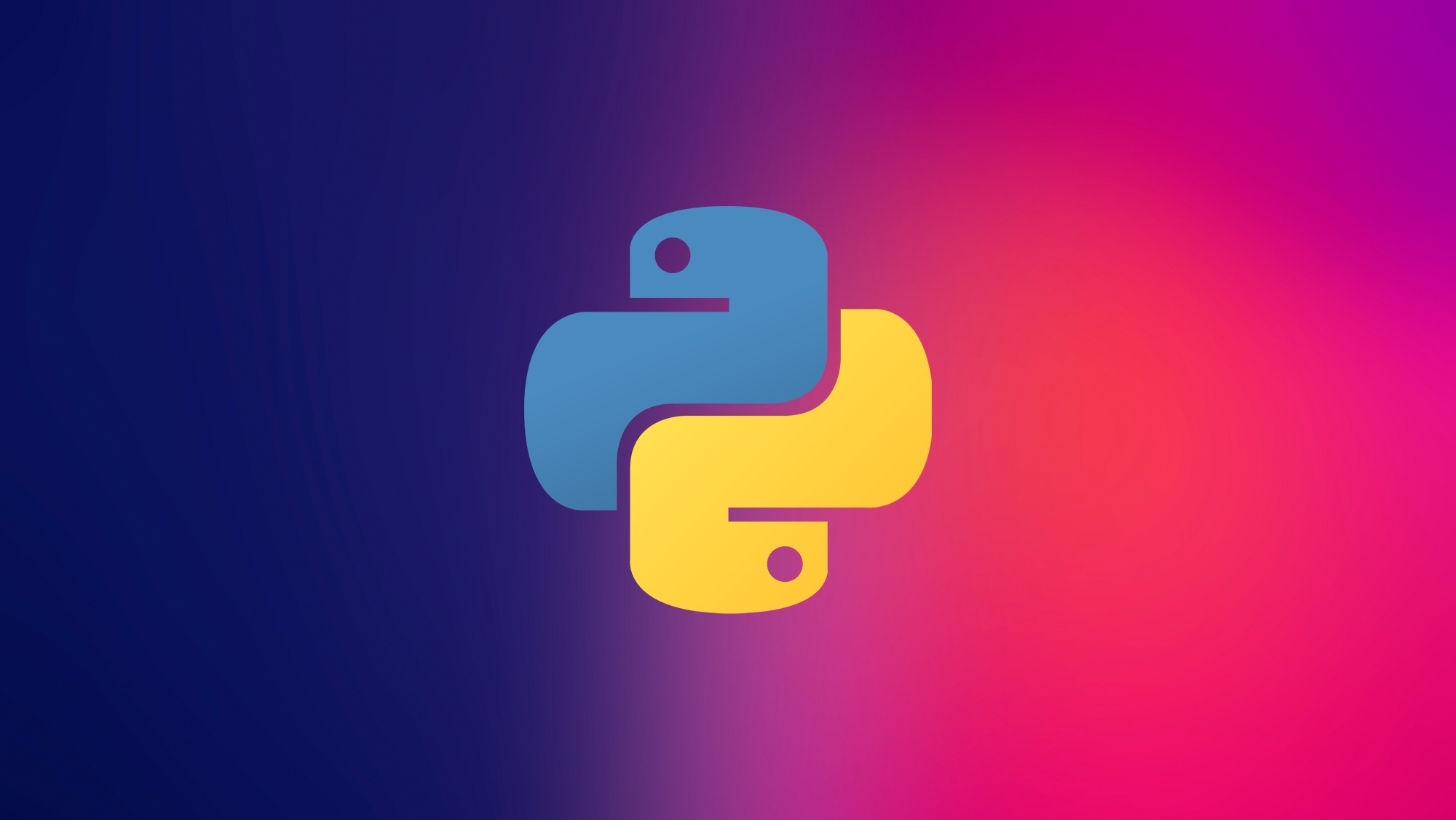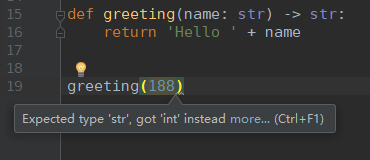# Python 类型标注VincentPython虽然简单，但也带来很多问题。尤其是弱类型一直被诟病，平时在写代码时，经常也会模糊参数的类型，导致debug难度增加。

## 常用的类型

from typing import List, Dict, Tuple

def greeting(name: str) -> str:    return 'Hello ' + name    print(greeting(188))def do_nothing(a: List[int], b: Tuple[int, str], c: Dict[str, int]) -> Dict[str, str]:    return {'key': 'value'}# 调用do_nothing(, (14, 'some'), {"ha": 10})

def do_nothing(a: Dict[str, List[Tuple[int, int, int]]], ):	pass

Point = Tuple[float, float, float]def compute_distance(p1: Point, points: List[Point]):    pass

## 多种类型

Union[int, str, float]

Union[str, None]# 或者Optional[str]

## 函数作为输入参数

def add_all(f: Callable[[int], int], params: List[int]):    return sum(map(f, params))print(add_all(lambda x: x**2, list(range(1, 10))))

## 返回生成器

from typing import List, Generatorimport mathdef get_data(l: List[int], num: int) -> Generator:    """    输入list， 每次按照num个数 返回数据块    :param l: list data    :param num: batch size    """    epochs = math.ceil(len(l) / num)    for epoch in range(epochs):        yield l[epoch * num:(epoch + 1) * num]for each in get_data(list(range(98)), 5):    print(each)

## 小结## 评论